# Word Space Models and Random Indexing

Oct. 10, 2014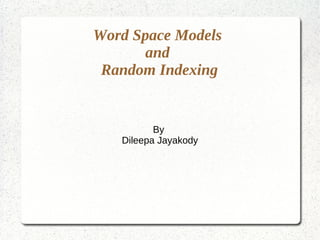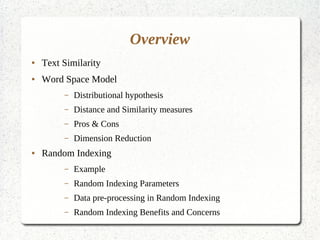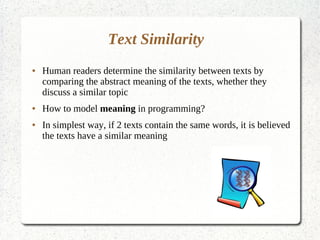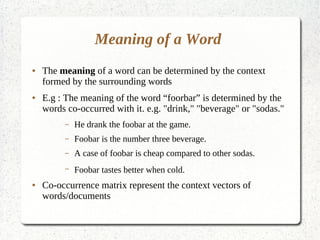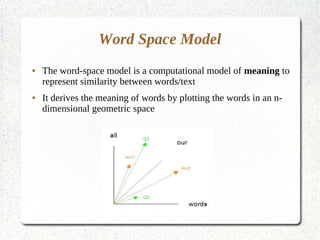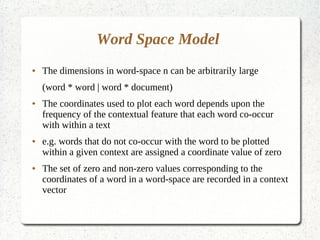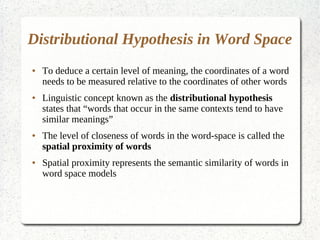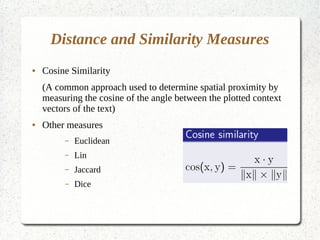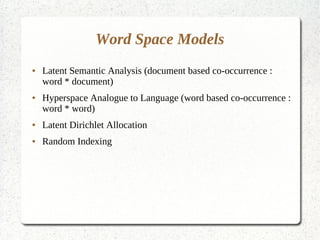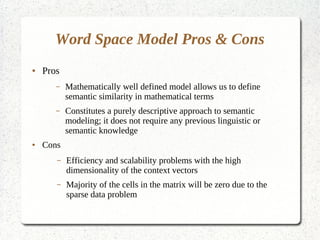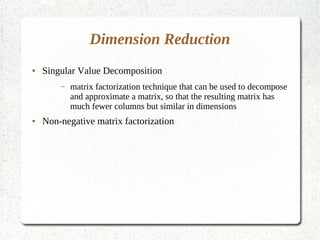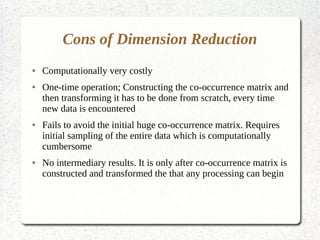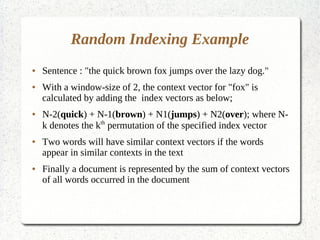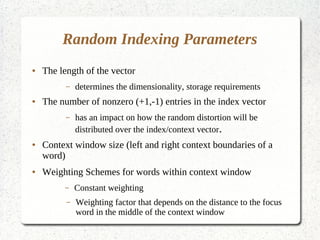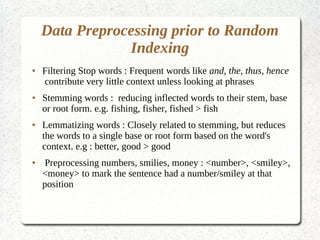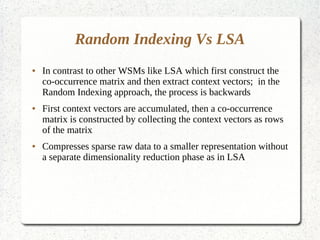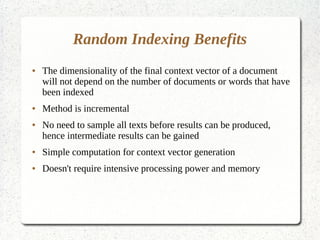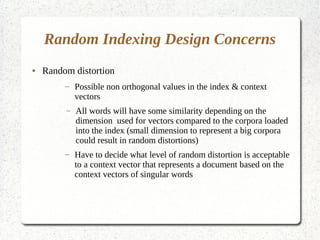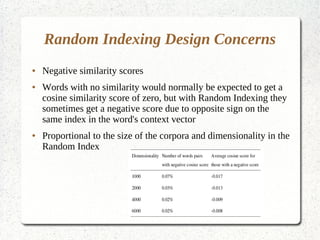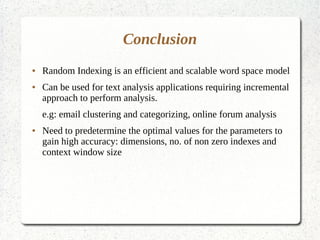1 of 22

### Word Space Models and Random Indexing

• 1. Word Space Models and Random Indexing By Dileepa Jayakody
• 2. Overview ● Text Similarity ● Word Space Model – Distributional hypothesis – Distance and Similarity measures – Pros & Cons – Dimension Reduction ● Random Indexing – Example – Random Indexing Parameters – Data pre-processing in Random Indexing – Random Indexing Benefits and Concerns
• 3. Text Similarity ● Human readers determine the similarity between texts by comparing the abstract meaning of the texts, whether they discuss a similar topic ● How to model meaning in programming? ● In simplest way, if 2 texts contain the same words, it is believed the texts have a similar meaning
• 4. Meaning of a Word ● The meaning of a word can be determined by the context formed by the surrounding words ● E.g : The meaning of the word “foorbar” is determined by the words co-occurred with it. e.g. "drink," "beverage" or "sodas." – He drank the foobar at the game. – Foobar is the number three beverage. – A case of foobar is cheap compared to other sodas. – Foobar tastes better when cold. ● Co-occurrence matrix represent the context vectors of words/documents
• 5. Word Space Model ● The word-space model is a computational model of meaning to represent similarity between words/text ● It derives the meaning of words by plotting the words in an n- dimensional geometric space
• 6. Word Space Model ● The dimensions in word-space n can be arbitrarily large (word * word | word * document) ● The coordinates used to plot each word depends upon the frequency of the contextual feature that each word co-occur with within a text ● e.g. words that do not co-occur with the word to be plotted within a given context are assigned a coordinate value of zero ● The set of zero and non-zero values corresponding to the coordinates of a word in a word-space are recorded in a context vector
• 7. Distributional Hypothesis in Word Space ● To deduce a certain level of meaning, the coordinates of a word needs to be measured relative to the coordinates of other words ● Linguistic concept known as the distributional hypothesis states that “words that occur in the same contexts tend to have similar meanings” ● The level of closeness of words in the word-space is called the spatial proximity of words ● Spatial proximity represents the semantic similarity of words in word space models
• 8. Distance and Similarity Measures ● Cosine Similarity (A common approach used to determine spatial proximity by measuring the cosine of the angle between the plotted context vectors of the text) ● Other measures – Euclidean – Lin – Jaccard – Dice
• 9. Word Space Models ● Latent Semantic Analysis (document based co-occurrence : word * document) ● Hyperspace Analogue to Language (word based co-occurrence : word * word) ● Latent Dirichlet Allocation ● Random Indexing
• 10. Word Space Model Pros & Cons ● Pros – Mathematically well defined model allows us to define semantic similarity in mathematical terms – Constitutes a purely descriptive approach to semantic modeling; it does not require any previous linguistic or semantic knowledge ● Cons – Efficiency and scalability problems with the high dimensionality of the context vectors – Majority of the cells in the matrix will be zero due to the sparse data problem
• 11. Dimension Reduction ● Singular Value Decomposition – matrix factorization technique that can be used to decompose and approximate a matrix, so that the resulting matrix has much fewer columns but similar in dimensions ● Non-negative matrix factorization
• 12. Cons of Dimension Reduction ● Computationally very costly ● One-time operation; Constructing the co-occurrence matrix and then transforming it has to be done from scratch, every time new data is encountered ● Fails to avoid the initial huge co-occurrence matrix. Requires initial sampling of the entire data which is computationally cumbersome ● No intermediary results. It is only after co-occurrence matrix is constructed and transformed the that any processing can begin
• 13. Random Indexing Magnus Sahlgren, Swedish Institute of Computer Science, 2005 ● A word space model that is inherently incremental and does not require a separate dimension reduction phase ● Each word is represented by two vectors – Index vector : contains a randomly assigned label. The random label is a vector filled mostly with zeros, except a handful of +1 and -1 that are located at random indexes. Index vectors are expected be orthogonal e.g. school = [0,0,0,......,0,1,0,...........,-1,0,..............] – Context vector : produced by scanning through the text; each time a word occurs in a context (e.g. in a document, or within a sliding context window), that context's d-dimensional index vector is added to the context vector of the word in question
• 14. Random Indexing Example ● Sentence : "the quick brown fox jumps over the lazy dog." ● With a window-size of 2, the context vector for "fox" is calculated by adding the index vectors as below; ● N-2(quick) + N-1(brown) + N1(jumps) + N2(over); where N- k denotes the kth permutation of the specified index vector ● Two words will have similar context vectors if the words appear in similar contexts in the text ● Finally a document is represented by the sum of context vectors of all words occurred in the document
• 15. Random Indexing Parameters ● The length of the vector – determines the dimensionality, storage requirements ● The number of nonzero (+1,-1) entries in the index vector – has an impact on how the random distortion will be distributed over the index/context vector. ● Context window size (left and right context boundaries of a word) ● Weighting Schemes for words within context window – Constant weighting – Weighting factor that depends on the distance to the focus word in the middle of the context window
• 16. Data Preprocessing prior to Random Indexing ● Filtering Stop words : Frequent words like and, the, thus, hence contribute very little context unless looking at phrases ● Stemming words : reducing inflected words to their stem, base or root form. e.g. fishing, fisher, fished > fish ● Lemmatizing words : Closely related to stemming, but reduces the words to a single base or root form based on the word's context. e.g : better, good > good ● Preprocessing numbers, smilies, money : <number>, <smiley>, <money> to mark the sentence had a number/smiley at that position
• 17. Random Indexing Vs LSA ● In contrast to other WSMs like LSA which first construct the co-occurrence matrix and then extract context vectors; in the Random Indexing approach, the process is backwards ● First context vectors are accumulated, then a co-occurrence matrix is constructed by collecting the context vectors as rows of the matrix ● Compresses sparse raw data to a smaller representation without a separate dimensionality reduction phase as in LSA
• 18. Random Indexing Benefits ● The dimensionality of the final context vector of a document will not depend on the number of documents or words that have been indexed ● Method is incremental ● No need to sample all texts before results can be produced, hence intermediate results can be gained ● Simple computation for context vector generation ● Doesn't require intensive processing power and memory
• 19. Random Indexing Design Concerns ● Random distortion – Possible non orthogonal values in the index & context vectors – All words will have some similarity depending on the dimension used for vectors compared to the corpora loaded into the index (small dimension to represent a big corpora could result in random distortions) – Have to decide what level of random distortion is acceptable to a context vector that represents a document based on the context vectors of singular words
• 20. Random Indexing Design Concerns ● Negative similarity scores ● Words with no similarity would normally be expected to get a cosine similarity score of zero, but with Random Indexing they sometimes get a negative score due to opposite sign on the same index in the word's context vector ● Proportional to the size of the corpora and dimensionality in the Random Index
• 21. Conclusion ● Random Indexing is an efficient and scalable word space model ● Can be used for text analysis applications requiring incremental approach to perform analysis. e.g: email clustering and categorizing, online forum analysis ● Need to predetermine the optimal values for the parameters to gain high accuracy: dimensions, no. of non zero indexes and context window size
• 22. Thank you